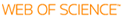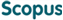BROWSE

Related ResearcherChoi, Su Jung
Research Interests
• Behavioral Finance
• Empirical Asset Pricing
• Investments

A fast algorithm to sample the number of vertexes and the area of the random convex hull on the unit square

Cited 0 times inCited 0 times inTitle
A fast algorithm to sample the number of vertexes and the area of the random convex hull on the unit square
Author
Issue Date
2014-10
Publisher
SPRINGER HEIDELBERG
Citation
COMPUTATIONAL STATISTICS, v.29, no.5, pp.1187 - 1205
Abstract
We propose an algorithm to sample the area of the smallest convex hull containing n sample points uniformly distributed over unit square. To do it, we introduce a new coordinate system for the position of vertexes and re-write joint distribution of the number of vertexes and their locations in the new coordinate system. The proposed algorithm is much faster than existing procedure and has a computational complexity on the order of O(T), where T is the number of vertexes. Using the proposed algorithm, we numerically investigate the asymptotic behavior of functionals of the random convex hull. In addition, we apply it to finding pairs of stocks where the returns are dependent on each other on the New York Stock Exchange.
URI
https://scholarworks.unist.ac.kr/handle/201301/10402
DOI
10.1007/s00180-014-0486-1
ISSN
0943-4062
Appears in Collections:
SBA_Journal Papers
Files in This Item: